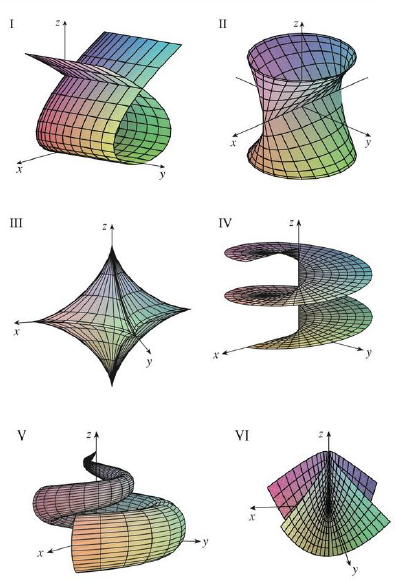Chapter 16.6, Problem 18E

Chapter
Section
Textbook Problem

Match the equations with the graphs labeled I–VI and give reasons for your answers. Determine which families of grid curves have u constant and which have v constant.18. x = sin u, y = cos u sin v, z = sin vTo determine

To match: The parametric equations x=sinu,y=sinusinv,z=sinv with the graphs labeled I-VI in the textbook and to explain the reason for the combination.

Explanation

Given data:

Write the given parametric equations as follows.

x=sinu,y=sinusinv,z=sinv (1)

The graphs are labeled in the textbook from I to VI.

Determination of grid curves when v is constant:

Consider the parameter v=v0 .

Substitute v0 for v in equation (1),

x=sinu,y=sinusinv0,z=sinv0

If the parameter v is held constant, the parameter z becomes constant.

The expression x=sinu,y=sinusinv0,z=sinv0 represents an ellipse that contains in the horizontal plane

Still sussing out bartleby?

Check out a sample textbook solution.

See a sample solution

The Solution to Your Study Problems

Bartleby provides explanations to thousands of textbook problems written by our experts, many with advanced degrees!

Get Started

Find the value of the sum. 24. k=08cosk

Single Variable Calculus: Early Transcendentals, Volume I

Simplify the expressions in Exercises 97106. (xy)1/3(yx)1/3

Finite Mathematics and Applied Calculus (MindTap Course List)

For the following set of scores, find the value of each expression: a. X b. (X)2 c. X2 d. (X +3)

Essentials of Statistics for The Behavioral Sciences (MindTap Course List)

In Problems 27-32, find the average value of each function over the given interval.

Mathematical Applications for the Management, Life, and Social Sciences

Evaluate the integral. 15. x1x2+2xdx

Single Variable Calculus: Early Transcendentals

True or False: If f′(c) = 0 and f″(c) < 0, then c is a local minimum.

Study Guide for Stewart's Single Variable Calculus: Early Transcendentals, 8th